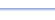Program: REMAINDR

REMAINDR

• Finds the remainder when a positive integer is divided by another positive integer.

Useful for:

• SAT
• ACT
• Algebra 1
• Algebra 2
• Basic Math

See full list of programs

Example

Q: Lights flash in the following pattern:  green, yellow, red, blue, green, yellow, red, blue, ...  What color is the 189th light?

Solution:

First, find the number of items in the pattern: 4.  Every fourth light will be blue, since it is the 4th color in the pattern.  Decide that you need to know the remainder when 189 is divided by 4, in order to answer the question.

Run REMAINDR.  How?

Enter
Number: 189
Divide by: 4

The program reports:

Interpretation:
Since the remainder is 1, the answer is the first item in the pattern: green.